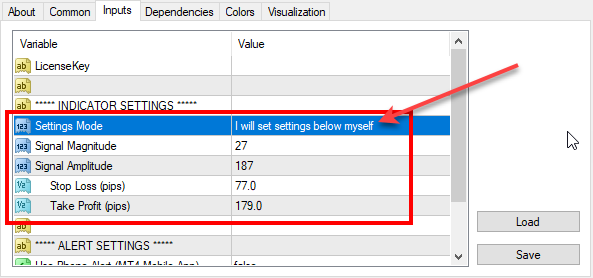# Re-optimized FxMagnetic indicator on GBPUSD (2018-09-17)

We’ve reoptimized FxMagnetic indicator settings on GBPUSD. Here are the latest parameters for all GBPUSD timeframes that you should use for best MT4 indicator performance.To set parameters manually you need to switch the Settings Mode to: I will set settings below myself

Don’t have FxMagnetic indicator yet? You can buy FxMagnetic here.

## 1-minute chart:

Signal Magnitude = 40

Signal Amplitude = 140

Stop Loss (pips) = 40

Take Profit (pips) = 85

## 5-minute chart:

Signal Magnitude = 20

Signal Amplitude = 130

Stop Loss (pips) = 70

Take Profit (pips) = 95

## 15-minute chart:

Signal Magnitude = 52

Signal Amplitude = 207

Stop Loss (pips) = 57

Take Profit (pips) = 102

## 30-minute chart:

Signal Magnitude = 27

Signal Amplitude = 187

Stop Loss (pips) = 79

Take Profit (pips) = 179

## 1-hour chart:

Signal Magnitude = 94

Signal Amplitude = 192

Stop Loss (pips) = 111

Take Profit (pips) = 131

## 4-hour chart:

Signal Magnitude = 4

Signal Amplitude = 103

Stop Loss (pips) = 100

Take Profit (pips) = 222

## Daily chart:

Signal Magnitude = 2

Signal Amplitude = 100

Stop Loss (pips) = 193

Take Profit (pips) = 235

## Weekly chart:

Signal Magnitude = 2

Signal Amplitude = 100

Stop Loss (pips) = 1500

Take Profit (pips) = 500

## Monthly chart:

Signal Magnitude = 2

Signal Amplitude = 100

Stop Loss (pips) = 1500

Take Profit (pips) = 1000